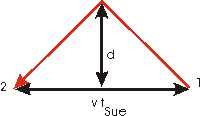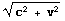# A Mathematical Supplement: Deriving Time Dilation

For Lou, we assume that the light source, light detector, and clock are all located in exactly the same position, which is not exactly the way the figure shows it. We will call the distance from the source to the mirror d.

The two events whose times of occurence Lou will measure are:

1. The light is emitted from the source
2. The light is detected by the detector

Since the light travels a total distance of 2d and travels at a speed c:

tLou = 2d / cSue observes the same two events, but relative to her the light source, mirror and detector are moving at some speed v to the left. We assume, correctly as it turns out, that the light travels a vertical distance d relative to Sue also. The horizontal distance between the two events relative to Sue is v tSue, as shown.

The total distance travelled by the light is:Note that the right hand side of the above equation uses the fact that the speed of light relative to Sue is also c. The equation can be rearranged to:which leads to the time dilation relation that we are deriving.

If it were not for Einstein's explanation of the Michelson-Morley experiment then if the speed of light relative to Lou were c, then the speed of that same light realtive to Sue would be:and the time between the two events as measured by Sue's clocks would be the same 2 d / c as measured by Lou's clocks.